# Ex 12.3 NCERT Solutions- Areas Related to Circles Class 10 Notes | EduRev

## Class 10 : Ex 12.3 NCERT Solutions- Areas Related to Circles Class 10 Notes | EduRev

The document Ex 12.3 NCERT Solutions- Areas Related to Circles Class 10 Notes | EduRev is a part of the Class 10 Course Class 10 Mathematics by VP Classes.
All you need of Class 10 at this link: Class 10

NCERT TEXTBOOK QUESTIONS SOLVED
Page No. 234
EXERCISE 12.3

Unless stated otherwise, use π = 22/7
Q 1. Find the area of the shaded region in Fig. PQ = 24 cm, PR = 7 cm and O is the centre of the circle.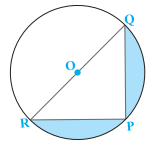Sol. Since O is the centre of the circle,
∴ QOR is a diameter.
⇒ ∠RPQ = 90° [Angle in a semi-circle]
Now, in right ∆ RPQ,
RQ2 = PQ2 + PR2
⇒ RQ2 = 242 + 72 = 576 + 49 = 625
⇒ RQ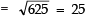∴ Area of Δ RPQ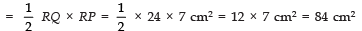Now, area of semi-circle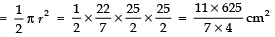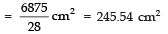∴ Area of the shaded portion = 245.54 cm2 − 84 cm2 = 161.54 cm2.

Page No. 235
Q 2. Find the area of the shaded region in figures, if radii of the two concentric circles with centre O are 7 cm and 14 cm respectively and ∠AOC = 40°.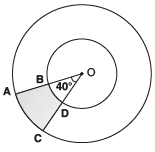Sol. Radius of the outer circle = 14 cm
Here, θ = 40°
∴ Area of the sector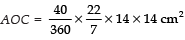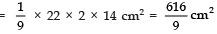Radius of the inner circle = 7 cm
Here, also θ = 40°
∴ Area of the sector BOD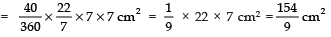Now, area of the shaded region = [Area of sector AOC] − [Area of sector BOD]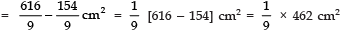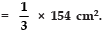Q 3. Find the area of the shaded region in figure, if ABCD is a square of side 14 cm and APD and BPC are semi-circles.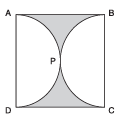Sol. Side of the square = 14 cm
∴ Area of the square ABCD=14 × 14 cm2 = 196 cm2
Now, diameter of the circle = (Side of the square) = 14 cm
⇒ Radius of each of the circles  = 14/2 = 7cm
∴ Area of the semi-circle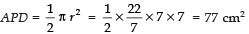Area of the semi-circle BPC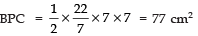∴ Area the shaded region = [Area of the square] − [Area of semicircle APD + Area of semicircle BPC]
= 196 − [77 + 77] cm2 = 196 − 154 cm2 = 42 cm2.

Q 4. Find the area of the shaded region in figure, where a circular arc of radius 6 cm has been drawn with vertex O of an equilateral triangle OAB of side 12 cm as centre.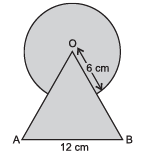Sol. Area of the circle with radius = 6 cm.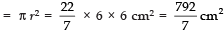Area of equilateral triangle, having side a = 12 cm, is given by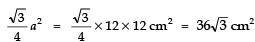∵ Each angle of an equilateral triangle = 60°
∠AOB = 60°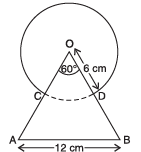∴ Area of sector COD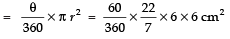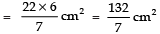Now, area of the shaded region, = [Area of the circle] + [Area of the equilateral triangle] − [Area of the sector COD]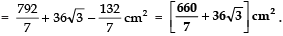Q 5. From each corner of a square of side 4 cm a quadrant of a circle of radius 1 cm is cut and also a circle of diameter 2 cm is cut as shown in figure. Find the area of the remaining portion of the square.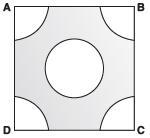Sol. Side of the square = 4 cm
∴ Area of the square ABCD = 4 × 4 cm2 = 16 cm2
∴ Area of all the 4 quadrant squares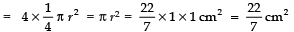Diameter of the middle circle = 2 cm
⇒ Radius of the middle circle = 1 cm
∴ Area of the middle circle = πr2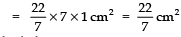Now, area of the shaded region = [Area of the square ABCD] − [(Area of the 4 quadrant circles) + (Area of the middle circle)]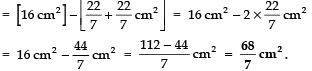Q 6. In a circular table cover of radius 32 cm, a design is formed leaving an equilateral triangle ABC in the middle as shown in figure. Find the area of the design.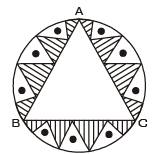Sol. Area of the circle having radius r = 32 cm.
= πr2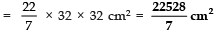‘O’ is the centre of the circle,
∴ AO = OB = OC = 32 cm
⇒ ∠AOB = ∠BOC = ∠AOC = 120°
Now, in Δ AOB, ∠1 = 30°
∵ ∠1 + ∠2 = 60°
Also OA = OB ⇒∠1 = ∠2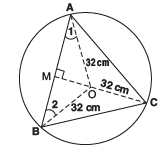If OM ⊥ AB, then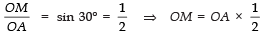⇒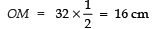Also,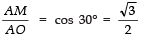⇒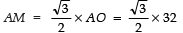⇒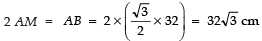Now, area of Δ AOB, =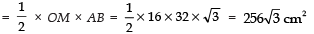Since area Δ ABC = 3 × [area of ∆ AOB] =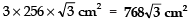Now, area of the design = [Area of the circle] − [Area of the equilateral triangle]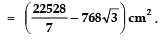Page No. 236
Q 7. In figure, ABCD is a square of side 14 cm. With centres A, B, C and D, four circles are drawn such that each circle touch externally two of the remaining three circles. Find the area of the shaded region.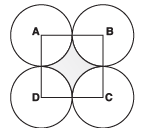Sol. Side of the square ABCD = 14 cm
∴ Area of the square ABCD = 14 × 14 cm2 = 196 cm2.
∵ Circles touch each other
∴ Radius of a circle = 14/2 = 7 cm
Now, area of a sector of radius 7 cm and sector angle θ as 90°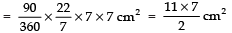⇒ Area of 4 sectors =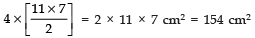∴ Area of the shaded region = [Area of the square ABCD] − [Area of the 4 sectors]
= 196 cm2 − 154 cm2 = 42 cm2.

Q 8. The figure depicts a racing track whose left and right ends are semicircular.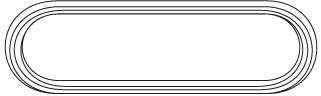The distance between the two inner parallel line segments is 60 m and they are each 106 m long.
If the track is 10 m wide, find:
(i) the distance around the track along its inner edge
(ii) the area of the track.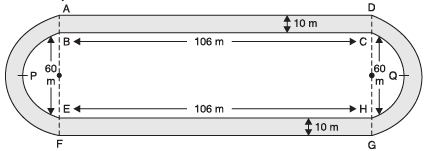Sol. (i) Distance around the track along its inner edge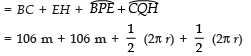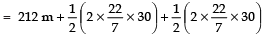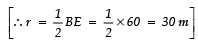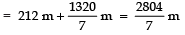(ii) Now, area of the track
= Area of the shaded region = (Area of rectangle ABCD) + (Area of rectangle EFGH)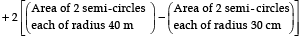[ The track is 10 m wide]
⇒ Area of the track
= (106 × 10 m2) + (106 × 10 m2)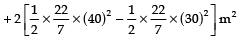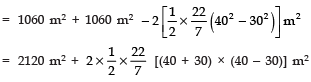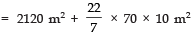= 2120 m2 + 2200 m2 = 4320 m2.

Q 9. In the figure, AB and CD are two diameters of a circle (with centre O) perpendicular to each other and OD is the diameter of the smaller circle. If OA = 7 cm, find the area of the shaded region.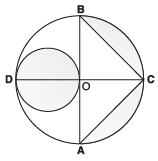Sol. O is the centre of the circle.
OA =7 cm
⇒ AB = 2 OA = 2 × 7 = 14 cm
OC = OA = 7 cm
∵ AB and CD are perpendicular to each other
⇒ OC ⊥ AB
∴ Area Δ ABC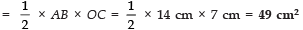Again OD = OA = 7 cm
∴ Radius of the small circle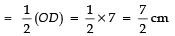∴ Area of the small circle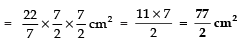Radius of the big circle  14/2 cm = 7 cm
∴ Area of semi-circle OABC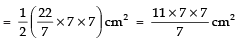= 11 × 7 cm2 = 77 cm2
Now, Area of the shaded region = [Area of the small circle] + [Area of the big semi-circle OABC] − [Area of Δ ABC]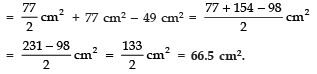Q 10. The area of an equilateral triangle ABC is 17320.5 cm2. With each vertex of the triangle as centre, a circle is drawn with radius equal to half the length of the side of the triangle (see Fig.). Find the area of the shaded region.
(Use π = 3.14 and √3 = 1.73205).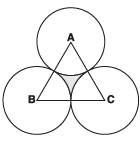Sol. Area of ∆ ABC = 17320.5 cm2
∵  Δ ABC is an equilateral triangle and area of an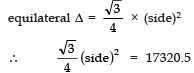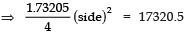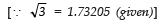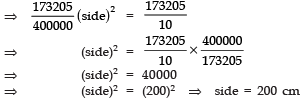⇒ Radius of each circle = 200/2 = 100 cm
Since each angle of an equilateral triangle is 60°,
∴∠A = ∠B = ∠C = 60°
∴ Area of a sector having angle of sector as 60° and radius 100 cm.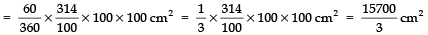∴ Area of 3 equal sectors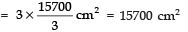Now, area of the shaded region = [Area of the equilateral triangle ABC] − [Area of 3 equal sectors]
= 17320.5 cm2 − 15700 cm2 = 1620.5 cm2.

Page No. 237
Q 11. On a square handkerchief, nine circular designs each of radius 7 cm are made (see figure). Find the area of the remaining portion of the handkerchief.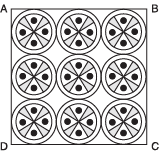Sol. ∵ The circles touch each other.
∴ The side of the square ABCD
= 3 × diameter of a circle
= 3 × (2 × radius of a circle) = 3 × (2 × 7 cm)
= 42 cm
⇒ Area of the square ABCD = 42 × 42 cm2 = 1764 cm2.
Now, area of one circle =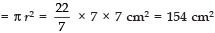∵ There are 9 squares
∴ Total area of 9 circles = 154 × 9 = 1386 cm2
∴ Area of the remaining portion of the handkerchief = 1764 − 1386 cm2 = 378 cm2.

Q 12. In the figure, OACB is a quadrant of a circle with centre O and radius 3.5 cm. If OD = 2 cm, find the area of the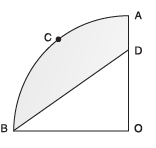Sol. Here, centre of the circle is O and radius = 3.5 cm.
∴ Area of the quadrant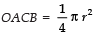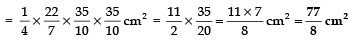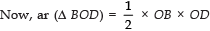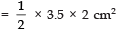[∵ OB = 3.5 cm = radius and OD = 2 cm (given)]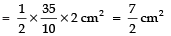∴ Area of the shaded region
= (Area of the quadrant OACB) − (Area of ∆ BOD)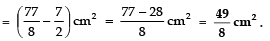Q 13. In the figure, a square OABC is inscribed in a quadrant OPBQ. If OA = 20 cm, find the area of the shaded region. (Use π = 3.14)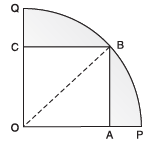Sol. Given: side of the square OABC = OA = 20 cm .
∴ Area of the square = 20 x 20 = 400 cm2
Diagonal of the square =√2 x (side of the square)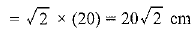Radius of the quadrant of circle = Diagonal of square = 20√2 cm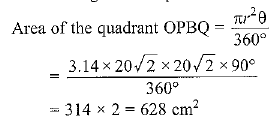Area of the shaded region = Area of the quadrant - Area of the square
=628 - 400 = 228 cm2

Q 14. AB and CD are respectively areas of two concentric circles of radii 21 cm and 7 cm and centre O (see figure). If ∠AOB = 30°, find the area of the shaded region.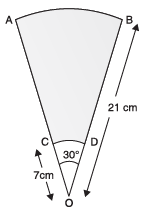Sol. ∵ Radius of bigger circle
R = 21 cm
and sector angle θ = 30°
∴ Area of the sector OAB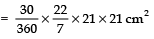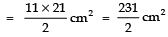Again, radius of the smaller circle r =7 cm
Here also, the sector angle is 30°
∴ Area of the sector OCD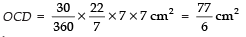∴ Area of the shaded region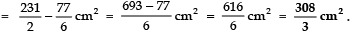Q 15. In the figure, ABPC is a quadrant of a circle of radius 14 cm and a semicircle is drawn with BC as diameter. Find the area of the shaded region.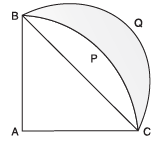Therefore, area of the quadrant ABPC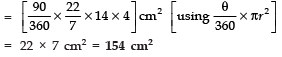Area of right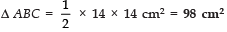⇒ Area of segment BPC = 154 cm2 − 98 cm2 = 56 cm2
Now, in right Δ ABC,
AC2 + AB2 = BC2
⇒ 142 + 142 = BC2
⇒ 196 + 196 = BC2
⇒ BC2 = 392 ⇒ BC = 14√2cm .
∴ Radius of the semi-circle BQC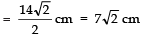∴ Area of the semi-circle BQC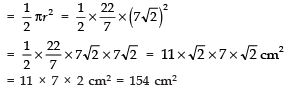Now, area of the shaded region = [Area of segment BQC] − [Area of segment BPC]
= 154 cm2 − 56 cm2 = 98 cm2.

Page No. 238
Q 16. Calculate the area of the designed region in the figure, common between the two quadrants of circles of radius 8 cm each.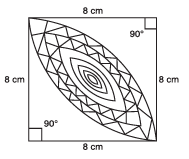Sol. ∵ Side of the square = 8 cm
∴ Area of the square (ABCD) = 8 × 8 cm2
= 64 cm2
∴ Area of the quadrant ADQB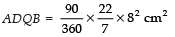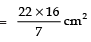Similarly, area of the quadrant BPDC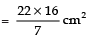∴ Sum of the two quadrants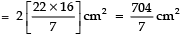Now, area of design = [Sum of the areas of the two quadrants] − [Area of the square ABCD]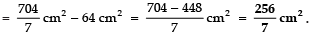Offer running on EduRev: Apply code STAYHOME200 to get INR 200 off on our premium plan EduRev Infinity!

132 docs

,

,

,

,

,

,

,

,

,

,

,

,

,

,

,

,

,

,

,

,

,

;